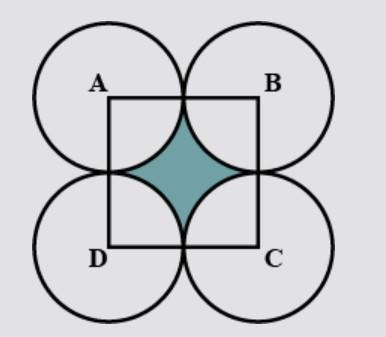QUESTION

# In the figure, ABCD is a square of side 14 cm. With centres A, B, C and D, four circles are drawn such that each circle touches externally two of the remaining two circles. Find the area of the shaded region.Hint: For solving this problem we should know how to calculate the area of square and circle. First, we will calculate the area of the square ABCD then we will subtract the area of 4 quadrants of each circle from the area of square ABCD to get the area of the shaded region.

Given:
We have to find the area of the shaded region shown in the figure below:It is given to us that the side of the square is 14 cm. Then,
$AB=BC=CD=DA=14\text{ cm}$

Now, the radius of each circle will be half of the length of the side of the square ABCD i.e. 7 cm.

Since, A, B, C and D are the centre of the four circles, there are four quadrants inside the square ABCD of each respective circle of radius 7 cm.

Now, we know that the area of the square of side $a$ cm is ${{a}^{2}}\text{ c}{{\text{m}}^{2}}$ . Then,
Area of the square ABCD $={{\left( 14 \right)}^{2}}=196\text{ c}{{\text{m}}^{2}}$ .

Now, we know that area of a circle of radius $r$ cm is $\pi {{r}^{2}}\text{ c}{{\text{m}}^{2}}$ . Then, the area of a quadrant of the circle will be $\dfrac{\pi {{r}^{2}}}{4}\text{ c}{{\text{m}}^{2}}$ . Then,
Area of one quadrant of a circle of radius 7 cm $=\dfrac{\pi \times {{7}^{2}}}{4}=\dfrac{49\pi }{4}\approx 38.4845\text{ c}{{\text{m}}^{2}}$ .

There are four such quadrants. So, the area of 4 quadrants will be 4 times the area of one quadrant.

Now, if we look at the figure below:From the above figure, we can say that,

Area of shaded region = (area of square) – (area of 4 quadrants).

Area of shaded region $=196-4\times 38.4845=42.062\text{ c}{{\text{m}}^{2}}$ .

Thus, the area of the shaded region will be $42.062\text{ c}{{\text{m}}^{2}}$ .

Note: Although the problem is very easy but one should take care while solving the question that, while calculating the area of the shaded region student must subtract the area of all 4 quadrants from the area of the square and avoid the calculation mistakes in the solution.